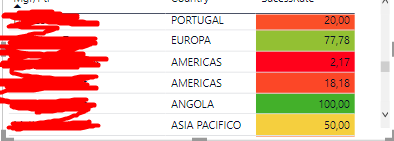cancel
Showing results for
Did you mean:New Member

## Multiply SucessRate per Country on each Person and Sum

Hello guys,

First post 😄

I'm having trouble on how to do this.

Context:

• There are contracts (each one with a  price) on Continents
• Persons have associated to them contracts
• Success Rate to each person depends on the totalContracts / ContractsWon percentage on each continent.

I have already implemented the Success RateSucess Rate Measure:

SucessRate 2 = (COUNTROWS(FILTER('Mgr/Ptr Sucess Rate'; 'Mgr/Ptr Sucess Rate'[WonOrNot] = 1))/ COUNTROWS('Mgr/Ptr Sucess Rate')) *100• First column:
• Sum of all contracts of Person X
• Second column:
• What I want to do:
• Multiply the success rate of the Person X on the Continent Y on the value of the contract that is on Continent Y
• What I have:
• ([SucessRate]/100) * CALCULATE(SUM('Manager Forecast'[MgrTotal]);'Manager Forecast'[Proposed] = 1)
• (Sum all the contracts and multiply by the success rate

I'm sorry if I didn't expose the situation correctly.

2 REPLIES 2Super User

Become an expert!: Enterprise DNA
External Tools: MSHGQM
Latest book!:
Mastering Power BI 2nd EditionDAX is easy, CALCULATE makes DAX hard...New Member

I'm sorry, is it better?